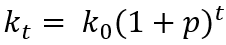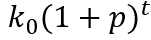Tackle 2024 with new knowledge – take advantage of Learnesys’ fall campaign on the All Courses package. Read more here.

# How to calculate interest on interest in Excel?

If you want to try the function FV() or the mathematical formula yourself, and/or reproduce the diagram below, there is a file for that here!

It will be reviewed in this article how to calculate interest on interest in Excel in two different ways. There is no generic function for this in Excel, you either have to rely on writing your own formula or use the FV() function. The effect of interest on interest is that you get interest on the interest that – for example – a saving has brought. The savings can increase in value both as interest on the capital and as interest on the previously paid interest.

## What is interest on interest?

Interest on interest, or compound interest, is interest calculated on the initial value of – for example – an investment, plus all previous interest during the period. Why you also sometimes talk about cumulative interest, i.e. interest on interest.

The purely mathematical formula for interest on interest looks like this:where p is the interest rate for a certain period (month, year etc), t is the number of periods and k0 is the initial value.

Examples of interest on interest effects are bank loans where the interest is usually paid several times a year. When the annual interest rate is divided by the number of periods per year, it has the effect that the effective interest rate is higher than the marketed annual interest rate. Another example is for investments where dividends are reinvested, which is why we get a similar effect.

Interest on interest is often mentioned together with the snowball effect. As a snowball grows and grows each time it is rolled in the snow, capital grows with each time period. If you start with a small snowball (small capital), it takes quite a long time at the beginning to make it grow, but gradually it will grow faster and faster. Let’s say you have \$10,000 that grows by 10% in the first year. This gives a return of \$1,000. If you choose to take that thousand, the money will continue to grow at the same rate year after year. If instead the return is allowed to remain, you will receive \$1,100 next year. We are talking here about exponential growth. The formula above is thus an exponential function, something that will be visualized later. To see how the formula works, you can insert values into it:The starting value is thus \$10,000, the interest rate p = 10% (the growth factor is 1.10) and year; t = 0, 1, …, 30.
10 000*(1+0,10)^0 10 000
10 000*(1+0,10)^1 11 000
10 000*(1+0,10)^2 12 100
¦ ¦
¦ ¦
10 000*(1+0,10)^30 174 494

This table provides the following chart:

The curve is thus a good example of the described snowball effect, i.e. the capital increases more drastically every year.

Exponential functions follow the same rules as all other functions. However, exponential functions have their own unique sub-rules. It is one of the most important functions in mathematics, and thus differs from perhaps the most important; the linear. In short, it can be said that it is the independent variable in the exponent that gives the curve its appearance.

If you have the graph, you can thus solve the equation “how many years will it take until I have saved this much?”. This can also be solved purely mathematically, but that will perhaps be a topic for a future article.

## The FV() function

The financial function FV() returns the future value of an investment based on a fixed interest rate. FV() can be used for fixed, recurring payments or lump sum payments.

FV() uses three required arguments, and two optional ones. This function with its arguments can be used to calculate the compound interest. I will show here how to calculate the compound interest spread over 30 years with four of the function’s five arguments, starting from the previous example:

=FV(rate,nper,pmt;[pv]) Result
See the first table and compare corresponding values.
=FV(0.1,0,0,-10000) \$10 000
=FV(0.1,1,0,-10000) \$11 000
=FV(0.1,2,0,-10000) \$12 100
¦ ¦
¦ ¦
=FV(0.1,30,0,-10000) \$174 494

This is consistent with the “theoretical” value. The function works like this:

`=FV(0,1;0;0;-10000)`

THE NAME OF THE FUNCTION (annual interest; number of periods where the return on the interest is to be added – in this case 1 – as it is annually; in this case number of years starting from 0; \$-10,000 is used as it is the investment that leaves the wallet).

As said, there is no generic formula for calculating interest on interest in Excel. FV() is usually used to calculate the final value of a loan, i.e. the amount of money you want to owe after all repayments have been made. This value should usually and reasonably be 0, since most people don’t want to incur any debt. The FV() function returns a loan’s final value if we have the other relevant values. Therefore, you can use the function to investigate what payment rate you should keep in order to be debt-free after x number of years.

It should also be said that FV() can be trickier to use in examples where periods are not years, but rather quarters or months. It is then necessary to think about how many payments you have to make per year. For monthly, the syntax goes something like this:

`=FV(0.10/12, number of years*12, 0, -10000),`

as we constantly have to take into account that there are 12 months in a year.

## How to calculate interest on interest mathematically in Excel

In Learnesy’s course The Excel Functions Guide, you learn around 100 useful Excel functions. However, a whole chapter is dedicated to financial functions – the functions FV() among other things. Likewise, there is a lesson for calculating interest on interest. In the lesson, the compound interest is calculated with a proprietary mathematical formula.

In the lesson, another example of a situation is brought up – but to return to the recurring example for this post, I will calculate the investment of \$10,000 after 30 years. Feel free to try this formula in the exercise file belonging to the lesson above. The formula (without any cell references) then becomes:

`=10000*(1+(0.1/1))^(1*30),`

which results in \$174,494. Feel free to try replacing the values in the cells instead. In the exercise file, you can also take the opportunity to use FV() in the same way as I have done in the table. Don’t forget to visualize this with a line or bar graph, or combine the two.

## Closing words

I hope this post will help you calculate interest on interest. It is relatively easy as long as you know the formula or you’re using the FV(). One should also understand what compound interest is.

If you want to know more about what courses Learnesy offers, you can do so here.

/Niklas, Learnesy

### More blog posts